# Hedge Algorithm

Hedge Algorithm is an algorithm for prediction with expert advice. Initially it was formulated for the different framework, than usual framework for the prediction game.

The outcome space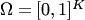. At each step gambler has a capital 1, which he distributes between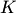of his friends in correspondence with fractions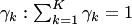. For each step each element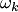of the oucome space is the loss of-th gambler's friend, if the gambler gave him all his money. Thus the loss of the gambler is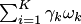. For the start of the game the weights are initialized by some probability distribution, like. For each step the protocol of the algorithm is the following for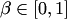:

- Receive vectorfrom the environment.
- Suffer loss.
- Set the new weights to be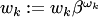, and normalize them.

In Freund and Schapire (1997, theorem 2) it is proven that for any sequence of outcomes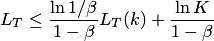for all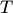and, where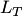is the loss suffered by the algorithm over the firsttrials, and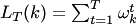is the loss (or expected loss) suffered by theth expert over the firsttrials.

The inequality above can be improved using Strong Aggregating Algorithm, as in Vovk (1998):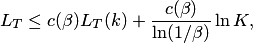where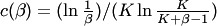. It is interesting whether it can be improved further.

The same method can be applied if the experts and the algorithm provide the probability distribution over the outcome space, and they suffer the expected loss of a decision randomly selected according to this distribution. In this case the algorithm predicts simply the weighted average of the experts predictions. In this case the loss function should be only bounded (one can compare with Weak Aggregating Algorithm). The theoretical bound for the loss of the Hedge algorithm is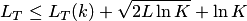for alland, whereis the loss (or expected loss) suffered by the algorithm over the firsttrials, and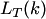is the loss (or expected loss) suffered by theth expert over the firsttrials. The constant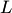is a prior upper bound on the loss of the best strategy, that in the worst case is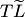, where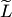is the bound for the loss used. This algorithm differs from the Weighted Average Algorithm by the learning rate for updating weights. The weights are updated by the rule: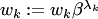, where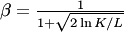, and then they are normalized. The bounds for some particular loss functions, like square-loss function, can be easily derived from the bound described above using geometrical inequalities.

### Bibliography

• Yoav Freund and Robert E. Schapire. A decision-theoretic generalization of on-line learning and an application to boosting. Journal of Computer and System Sciences, 55(1):119--139, (1997).
• Vladimir Vovk. A game of prediction with expert advice. Journal of Computer and System Sciences, 56:153 - 173, 1998.